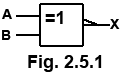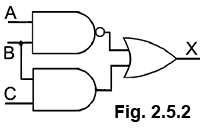# Digital Logic Quiz

Try our quiz, based on the information you can find in Digital Electronics Module 2 − Digital Logic. Submit your answers and see how many you get right. If you get any answers wrong. Just follow the hints to find the right answer and learn about the number systems used in digital electronics as you go.

### 1.What is the device illustrated in Fig.2.5.1?

### 2.Which of the following Boolean equations describes the action of Fig. 2.5.2?

### 3.

Which Boolean law is described by the equation A•(B+C) = A•B+A•C ?

### 4.Which of the following logic functions is illustrated by Fig. 2.5.3?

### 5.Which of the following Boolean equations describes the truth table in Fig. 2.5.4?

### 6.What type of I.C does Fig. 2.5.5 represent?

### 7.Which logic function does the circuit in Fig. 2.5.6 perform?

### 8.What is the Boolean expression that can be obtained for the Karnaugh map cell indicated in Fig. 2.5.7?

### 9.

Refer to Fig. 2.5.7. What is the minimum number of cell groups that can be obtained from the Karnaugh map using logic 1 cells only?

### 10.

Which of the segments on a 7 segment LED display need to be illuminated to display the decimal number 4?

Top of Page